The graph which represent the variation of mean kinetic energy of molecules with temperature t°C is

1.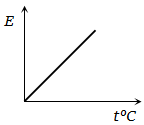2.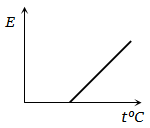3.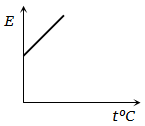4.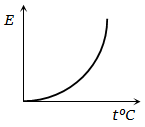High Yielding Test Series + Question Bank - NEET 2020

Difficulty Level:

The adjoining figure shows graph of pressure and volume of a gas at two temperatures ${\mathrm{T}}_{1}$ and ${\mathrm{T}}_{2}$. Which of the following interferences is correct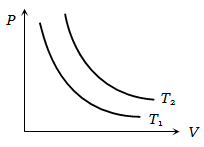1.  ${\mathrm{T}}_{1}>{\mathrm{T}}_{2}$

2. ${\mathrm{T}}_{1}={\mathrm{T}}_{2}$

3. ${\mathrm{T}}_{1}<{\mathrm{T}}_{2}$

4. No interference can be drawn

High Yielding Test Series + Question Bank - NEET 2020

Difficulty Level:

The expansion of until mass of a perfect gas at constant pressure is shown in the diagram. Here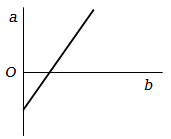1. a = volume, b = $°\mathrm{C}$ temperature

2. a = volume, b = $\mathrm{K}$ temperature

3. a = $°\mathrm{C}$ temperature, b = volume

4. a = $\mathrm{K}$ temperature, b = volume

High Yielding Test Series + Question Bank - NEET 2020

Difficulty Level:

An ideal gas is initially at temperature T and volume V. Its volume is increased by $∆\mathrm{V}$ due to an increase in temperature $∆\mathrm{T}$, pressure remaining constant. The quantity $\mathrm{\delta }=∆\mathrm{V}/\left(\mathrm{V}∆\mathrm{T}\right)$ varies with temperature as

1.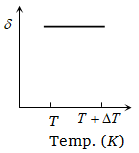2.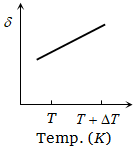3.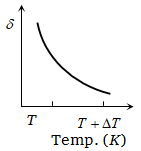4.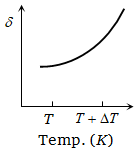High Yielding Test Series + Question Bank - NEET 2020

Difficulty Level:

Pressure versus temperature graph of an ideal gas of equal number of moles of different volumes are plotted as shown in figure. Choose the correct alternative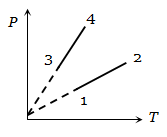1.

2.

3.

4.

High Yielding Test Series + Question Bank - NEET 2020

Difficulty Level:

Pressure versus temperature graph of an ideal gas is as shown in figure. Density of the gas at point A is ${\mathrm{\rho }}_{0}$ . Density at B will be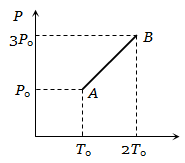1.  $\frac{3}{4}{\mathrm{\rho }}_{0}$

2.  $\frac{3}{2}{\mathrm{\rho }}_{0}$

3.  $\frac{4}{3}{\mathrm{\rho }}_{0}$

4.

High Yielding Test Series + Question Bank - NEET 2020

Difficulty Level:

The figure shows graphs of pressure versus density for an ideal gas at two temperatures ${\mathrm{T}}_{1}$ and ${\mathrm{T}}_{2}$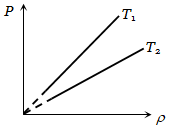1. ${\mathrm{T}}_{1}>{\mathrm{T}}_{2}$

2. ${\mathrm{T}}_{1}={\mathrm{T}}_{2}$

3. ${\mathrm{T}}_{1}<{\mathrm{T}}_{2}$

4. Nothing can be predicted

High Yielding Test Series + Question Bank - NEET 2020

Difficulty Level:

Graph of specific heat at constant volume for a monoatomic gas is

1.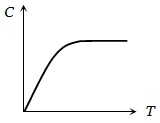2.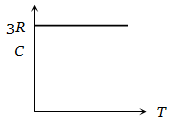3.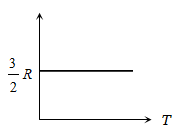4.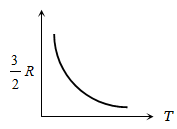High Yielding Test Series + Question Bank - NEET 2020

Difficulty Level:

A fix amount of nitrogen gas (1 mole) is taken and is subjected to pressure and temperature variation. The experiment is performed at high pressure as well as high temperatures. The results obtained are shown in the figures. The correct variation of PV/RT with P will be exhibited by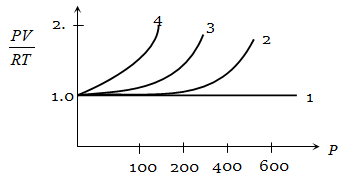1.  4

2.  3

3.  2

4.  1

High Yielding Test Series + Question Bank - NEET 2020

Difficulty Level:

A pressure P - absolute temperature T diagram was obtained when a given mass of gas was heated. During the heating process from the state 1 to state 2 the volume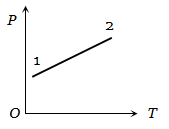1. Remained constant

2. Decreased

3. Increased

4. Changed erratically